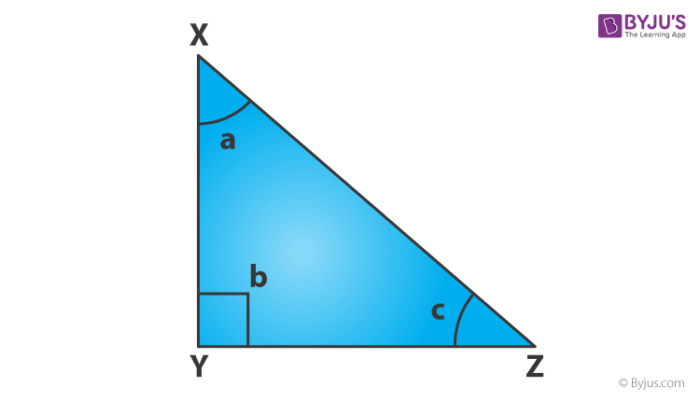How is sin calculated

In trigonometry, sine is one of three primary functions. Sine function is abbreviated as sin. To calculate the sine of an angle we have to know the length of the side opposite to the angle and hypotenuse of the right triangle.

Sin (Angle) = Side opposite to angle/Hypotenuse side

In a right-angled triangle, sine function is defined as the ratio of opposite side and hypotenuse, with respect to the angle.

Suppose, a right triangle XYZ as given below:Therefore, from the above figure, we can find sin of angle c and a such that;

Sin c = XY/XZ

Sin a = ZY/XZ(0)(0)